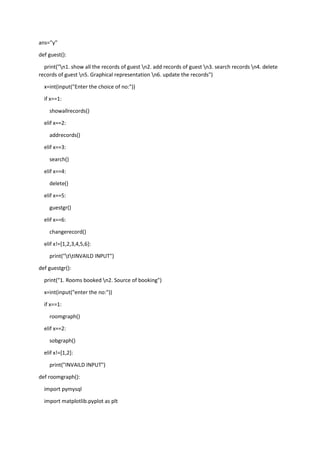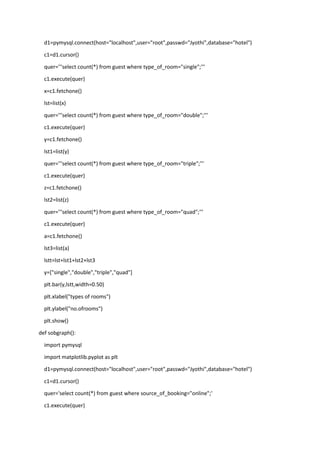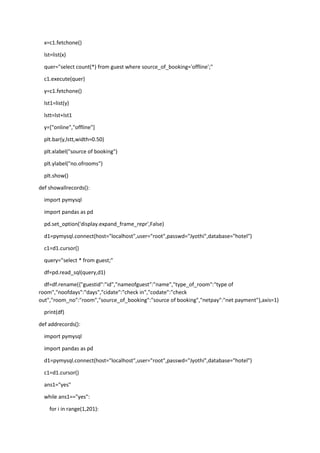Successfully reported this slideshow.

# hotelmangt project code connecting python wih Mysql cbse 2022-23 project .docx×

# hotelmangt project code connecting python wih Mysql cbse 2022-23 project .docx

do connect pytho with mysql
code :-
import mysql.connector
mydb = mysql.connector.connect(
host="localhost",
port = 8888, #for Mamp users
database='whatever db you want'
)
print(mydb)

do connect pytho with mysql
code :-
import mysql.connector
mydb = mysql.connector.connect(
host="localhost",
port = 8888, #for Mamp users
database='whatever db you want'
)
print(mydb)

### hotelmangt project code connecting python wih Mysql cbse 2022-23 project .docx

1. 1. ans="y" def guest(): print("n1. show all the records of guest n2. add records of guest n3. search records n4. delete records of guest n5. Graphical representation n6. update the records") x=int(input("Enter the choice of no:")) if x==1: showallrecords() elif x==2: addrecords() elif x==3: search() elif x==4: delete() elif x==5: guestgr() elif x==6: changerecord() elif x!=[1,2,3,4,5,6]: print("ttINVAILD INPUT") def guestgr(): print("1. Rooms booked n2. Source of booking") x=int(input("enter the no:")) if x==1: roomgraph() elif x==2: sobgraph() elif x!=[1,2]: print("INVAILD INPUT") def roomgraph(): import pymysql import matplotlib.pyplot as plt
2. 2. d1=pymysql.connect(host="localhost",user="root",passwd="Jyothi",database="hotel") c1=d1.cursor() quer='''select count(*) from guest where type_of_room="single";''' c1.execute(quer) x=c1.fetchone() lst=list(x) quer='''select count(*) from guest where type_of_room="double";''' c1.execute(quer) y=c1.fetchone() lst1=list(y) quer='''select count(*) from guest where type_of_room="triple";''' c1.execute(quer) z=c1.fetchone() lst2=list(z) quer='''select count(*) from guest where type_of_room="quad";''' c1.execute(quer) a=c1.fetchone() lst3=list(a) lstt=lst+lst1+lst2+lst3 y=["single","double","triple","quad"] plt.bar(y,lstt,width=0.50) plt.xlabel("types of rooms") plt.ylabel("no.ofrooms") plt.show() def sobgraph(): import pymysql import matplotlib.pyplot as plt d1=pymysql.connect(host="localhost",user="root",passwd="Jyothi",database="hotel") c1=d1.cursor() quer='select count(*) from guest where source_of_booking="online";' c1.execute(quer)
3. 3. x=c1.fetchone() lst=list(x) quer="select count(*) from guest where source_of_booking='offline';" c1.execute(quer) y=c1.fetchone() lst1=list(y) lstt=lst+lst1 y=["online","offline"] plt.bar(y,lstt,width=0.50) plt.xlabel("source of booking") plt.ylabel("no.ofrooms") plt.show() def showallrecords(): import pymysql import pandas as pd pd.set_option('display.expand_frame_repr',False) d1=pymysql.connect(host="localhost",user="root",passwd="Jyothi",database="hotel") c1=d1.cursor() query="select * from guest;" df=pd.read_sql(query,d1) df=df.rename({"guestid":"id","nameofguest":"name","type_of_room":"type of room","noofdays":"days","cidate":"check in","codate":"check out","room_no":"room","source_of_booking":"source of booking","netpay":"net payment"},axis=1) print(df) def addrecords(): import pymysql import pandas as pd d1=pymysql.connect(host="localhost",user="root",passwd="Jyothi",database="hotel") c1=d1.cursor() ans1="yes" while ans1=="yes": for i in range(1,201):
4. 4. x=i quer2="select * from guest where guestid=%d" %x c1.execute(quer2) if c1.rowcount==0: ans1="no" break y=input("Enter the guest name:") an="yes" while an=="yes": v=input("enter the type of room:") if v=="single": an="no" elif v=="double": an="no" elif v=="triple": an="no" elif v=="quad": an="no" else: print("invaild input") r=int(input("enter the no. of days:")) ci=input("enter the check in date:") co=input("entr the check out date:") ans3="yes" while ans3=="yes": a=input("Enter the source of booking:") if a=="offline": ans3="nooo" elif a=="online": ans3="nooo" else:
5. 5. print("invaild input") ans2="ye" if v=="single": while ans2=="ye": for i in range(1,51): c=i quer2="select * from guest where room_no=%d" %c c1.execute(quer2) if c1.rowcount==0: ans2="no" break elif v=="double": while ans2=="ye": for i in range(51,101): c=i quer2="select * from guest where room_no=%d" %c c1.execute(quer2) if c1.rowcount==0: ans2="no" break elif v=="triple": while ans2=="ye": for i in range(101,151): c=i quer2="select * from guest where room_no=%d" %c c1.execute(quer2) if c1.rowcount==0: ans2="no" break elif v=="quad": while ans2=="ye":
6. 6. for i in range(151,201): c=i quer2="select * from guest where room_no=%d" %c c1.execute(quer2) if c1.rowcount==0: ans2="no" break if v=="single": b=2000*r+(2000*r*9/50) elif v=="double": b=4000*r+(4000*r*9/50) elif v=="triple": b=6000*r+(6000*r*9/50) elif v=="quad": b=8000*r+(8000*r*9/50) quer="Insert into guest values(%d,'%s','%s',%d,'%s','%s',%d,'%s',%d);" %(x,y,v,r,ci,co,c,a,b) c1.execute(quer) d1.commit() print("Record Added") f=input("Want to see the added record:") if f=="y": pd.set_option('display.expand_frame_repr',False) quer="select * from guest where guestid=%d;"%x df=pd.read_sql(quer,d1) df1=df.rename({"guestid":"id","nameofguest":"name","type_of_room":"type of room","noofdays":"days","cidate":"check in","codate":"check out","room_no":"room","source_of_booking":"source of booking","netpay":"net payment"},axis=1) print(df1) else: print("Thank You") def search(): import pymysql
7. 7. import pandas as pd d1=pymysql.connect(user="root",host="localhost",passwd="Jyothi",database="hotel") c1=d1.cursor() print("1. Id n2. Name n3. Source of booking n4. Room no. n5. date n6. Type of room") cho=int(input("enter the no.")) if cho==1: pd.set_option('display.expand_frame_repr',False) x=int(input("enter the id:")) quer="select * from guest where guestid='%d';" %x df=pd.read_sql(quer,d1) df1=df.rename({"guestid":"id","nameofguest":"name","type_of_room":"type of room","noofdays":"days","cidate":"check in","codate":"check out","room_no":"room","source_of_booking":"source of booking","netpay":"net payment"},axis=1) print(df1) elif cho==2: pd.set_option('display.expand_frame_repr',False) x=input("enter the name:") quer="select * from guest where nameofguest='%s';" %x df=pd.read_sql(quer,d1) df1=df.rename({"guestid":"id","nameofguest":"name","type_of_room":"type of room","noofdays":"days","cidate":"check in","codate":"check out","room_no":"room","source_of_booking":"source of booking","netpay":"net payment"},axis=1) print(df1) elif cho==3: pd.set_option('display.expand_frame_repr',False) x=input("enter the source of booking:") quer="select * from guest where source_of_booking='%s';" %x df=pd.read_sql(quer,d1) df1=df.rename({"guestid":"id","nameofguest":"name","type_of_room":"type of room","noofdays":"days","cidate":"check in","codate":"check out","room_no":"room","source_of_booking":"source of booking","netpay":"net payment"},axis=1) print(df1) elif cho==4:
8. 8. pd.set_option('display.expand_frame_repr',False) x=int(input("enter the room no:")) quer="select * from guest where room_no='%d';" %x df=pd.read_sql(quer,d1) df1=df.rename({"guestid":"id","nameofguest":"name","type_of_room":"type of room","noofdays":"days","cidate":"check in","codate":"check out","room_no":"room","source_of_booking":"source of booking","netpay":"net payment"},axis=1) print(df1) elif cho==5: pd.set_option('display.expand_frame_repr',False) x=input("enter the date:") quer="select * from guest where cidate='%s';" %x df=pd.read_sql(quer,d1) df1=df.rename({"guestid":"id","nameofguest":"name","type_of_room":"type of room","noofdays":"days","cidate":"check in","codate":"check out","room_no":"room","source_of_booking":"source of booking","netpay":"net payment"},axis=1) print(df1) elif cho==6: pd.set_option('display.expand_frame_repr',False) x=input("enter the type of room:") quer="select * from guest where type_of_room='%s'" %x df=pd.read_sql(quer,d1) df1=df.rename({"guestid":"id","nameofguest":"name","type_of_room":"type of room","noofdays":"days","cidate":"check in","codate":"check out","room_no":"room","source_of_booking":"source of booking","netpay":"net payment"},axis=1) print(df1) def delete(): import pymysql d1=pymysql.connect(host="localhost",user="root",passwd="Jyothi",database="hotel") c1=d1.cursor() x=int(input("enter the id:")) quer="delete from guest where guestid=%d;" %x rowcount=c1.execute(quer)
9. 9. if rowcount>0: d1.commit() print("Record Deleted") else: print("NO RECORD FOUND") def changerecord(): import pymysql import pandas as pd pd.set_option('display.expand_frame_repr',False) d1=pymysql.connect(user="root",host="localhost",passwd="Jyothi",database="hotel") c1=d1.cursor() guid=int(input("enter the id:")) quer="select * from guest where guestid=%d" % guid c1.execute(quer) if c1.rowcount>0: row=list(c1.fetchone()) df=pd.read_sql(quer,d1) print(df) print("n1. nameofguest n2. source of booking n3. date n4. type of room") cr=int(input("enter the no:")) if cr==1: y=input("enter the new name of guest:") quer="update guest set nameofguest='%s' where guestid=%d" %(y,guid) c1.execute(quer) d1.commit() print("RECORD CHANGED") elif cr==2: y=input("enter the new source guest:") quer="update guest set source_of_booking='%s' where guestid=%d" %(y,guid) c1.execute(quer) d1.commit()
10. 10. print("RECORD CHANGED") elif cr==3: y=input("enter the new date:") quer="update guest set cidate='%s' where guestid=%d" %(y,guid) c1.execute(quer) d1.commit() print("RECORD CHANGED") elif cr==4: y=input("enter the new type of room:") quer="update guest set type_of_room='%s' where guestid=%d" %(y,guid) c1.execute(quer) d1.commit() quer4="select nofdays from guest where guestid=%d" %(guid) c1.execute(quer4) r=list(c1.fetchone()) ans2="ye" if y=="single": while ans2=="ye": for i in range(1,51): c=i quer2="select * from guest where room_no=%d" %c c1.execute(quer2) if c1.rowcount==0: ans2="no" break elif y=="double": while ans2=="ye": for i in range(51,101): c=i quer2="select * from guest where room_no=%d" %c c1.execute(quer2)
11. 11. if c1.rowcount==0: ans2="no" break elif y=="triple": while ans2=="ye": for i in range(101,151): c=i quer2="select * from guest where room_no=%d" %c c1.execute(quer2) if c1.rowcount==0: ans2="no" break elif y=="quad": while ans2=="ye": for i in range(151,201): c=i quer2="select * from guest where room_no=%d" %c c1.execute(quer2) if c1.rowcount==0: ans2="no" break quer1="update guest set room_no='%d' where guestid=%d" %(c,guid) c1.execute(quer1) d1.commit() if y=="single": b=2000*r+(2000*r*9/50) elif y=="double": b=4000*r+(4000*r*9/50) elif y=="triple": b=6000*r+(6000*r*9/50) elif y=="quad":
12. 12. b=8000+r+(8000*r*9/50) quer2="update guest set netpay=%d where guestid=%d" %(b,guid) c1.execute(quer2) d1.commit() print("RECORD CHANGED") elif cr!=[1,2,3,4,5,6,7]: print("INVAILD INPUT") elif c1.rowcount==0: print("NO RECORD FOUND TO CHANGE") def staff(): print("n1. show all the records of staff n2. add records of staffn3. search records of staff n4. delete records of staff n5. Graphical representation n6. update the records") x=int(input("Enter the choice of no.")) if x==1: allrecords() elif x==2: addrecordss() elif x==3: searchrec() elif x==4: deleterec() elif x==5: staffgr() elif x==6: changerec() elif x!=[1,2,3,4,5,6]: print("ttINVAILD INPUT") def staffgr(): print("1. Department n2. Salary") x=int(input("enter the no:")) if x==1:
13. 13. deptgraph() elif x==2: salgraph() def deptgraph(): import pymysql import matplotlib.pyplot as plt d1=pymysql.connect(host="localhost",user="root",passwd="Jyothi",database="hotel") c1=d1.cursor() quer="select count(*) from staff where dept='managment';" c1.execute(quer) x=c1.fetchone() lst=list(x) quer="select count(*) from staff where dept='cleaning';" c1.execute(quer) y=c1.fetchone() lst1=list(y) quer="select count(*) from staff where dept='food and beverages';" c1.execute(quer) z=c1.fetchone() lst2=list(z) lstt=lst+lst1+lst2 y=["managment","cleaning","food & beverages"] plt.bar(y,lstt) plt.xlabel("department") plt.ylabel("no.ofstaff") plt.show() def salgraph(): import pymysql import matplotlib.pyplot as plt d1=pymysql.connect(host="localhost",user="root",passwd="Jyothi",database="hotel") c1=d1.cursor()
14. 14. quer="select count(*) from staff where sal=2000;" c1.execute(quer) x=c1.fetchone() lst=list(x) quer="select count(*) from staff where sal=4000;" c1.execute(quer) y=c1.fetchone() lst1=list(y) quer="select count(*) from staff where sal=6000;" c1.execute(quer) z=c1.fetchone() lst2=list(z) lstt=lst+lst1+lst2 y=["2000","4000","6000"] plt.bar(y,lstt) plt.xlabel("salary") plt.ylabel("no.ofstaff") plt.show() def addrecordss(): import pymysql d1=pymysql.connect(host="localhost",user="root",passwd="Jyothi",database="hotel") c1=d1.cursor() print("n1. cleaning n2. food and beverages n3. managment") print("") ans1="yes" while ans1=="yes": for i in range(1,201): x=i quer2="select * from staff where id=%d" %x c1.execute(quer2) if c1.rowcount==0:
15. 15. ans1="no" break y=input("Enter the name:") a=input("Enter the department:") if a=="cleaning": j=2000 elif a=="food and beverages": j=4000 elif a=="managment": j=6000 z=input("Enter the hiredate:") quer="Insert into staff values(%d,'%s','%s',%d,'%s');" %(x,y,a,j,z) c1.execute(quer) d1.commit() print("Record Added") f=input("Want to see the added record:") if f=="y": quer="select * from staff where id=%d;"%x c1.execute(quer) rec=c1.fetchone() sid,sname,dept,sal,Hdate=rec print("staff id= %d"%sid,"staff name= %s"%sname,"depatment= %s"%dept,"salary= %d"%sal,"hireDate= %s"%Hdate,sep="n") else: print("THANK YOU") def deleterec(): import pymysql d1=pymysql.connect(host="localhost",user="root",passwd="Jyothi",database="hotel") c1=d1.cursor() x=int(input("enter the id:")) quer="delete from staff where id=%d;" %x
16. 16. rowcount=c1.execute(quer) if rowcount>0: d1.commit() print("Record Deleted") else: print("NO RECORD FOUND") def searchrec(): import pymysql import pandas as pd d1=pymysql.connect(host="localhost",user="root",passwd="Jyothi",database="hotel") c1=d1.cursor() print("n1. id n2. name n3. dept n4. salary n5. hiredate") cho=int(input("enter the no.")) if cho==1: pd.set_option('display.expand_frame_repr',False) x=int(input("enter the id:")) quer="select * from staff where id='%d';" %x df=pd.read_sql(quer,d1) print(df) elif cho==2: pd.set_option('display.expand_frame_repr',False) x=input("enter the name:") quer="select * from staff where name='%s';" %x df=pd.read_sql(quer,d1) print(df) elif cho==3: pd.set_option('display.expand_frame_repr',False) x=input("enter the dept:") quer="select * from staff where dept='%s';" %x df=pd.read_sql(quer,d1) print(df)
17. 17. elif cho==4: pd.set_option('display.expand_frame_repr',False) x=int(input("enter the salary:")) quer="select * from staff where sal=%d;" %x df=pd.read_sql(quer,d1) print(df) elif cho==5: pd.set_option('display.expand_frame_repr',False) x=input("enter the hire date:") quer="select * from staff where hiredate='%s';" %x df=pd.read_sql(quer,d1) print(df) elif cho!=[1,2,3,4,5]: print("invaild input") def changerec(): import pymysql import pandas as pd d1=pymysql.connect(user="root",host="localhost",passwd="Jyothi",database="hotel") c1=d1.cursor() sid=int(input("enter the id:")) quer="select * from staff where id=%d" % sid dfgg=pd.read_sql(quer,d1) print(dfgg) c1.execute(quer) if c1.rowcount>0: print("1. id n2. name n3. department n4. hire date") cr=int(input("enter the no:")) if cr==1: ans1="yes" while ans1=="yes": y=int(input("enter the id:"))
18. 18. quer1="select * from staff where id=%d" %y c1.execute(quer1) if c1.rowcount>0: print("DUPLICATE INPUT") elif c1.rowcount==0: ans1="no" quer="update staff set id=%d where id=%d" %(y,sid) c1.execute(quer) d1.commit() print("RECORD UPDATED") elif cr==2: y=input("enter the name:") quer="update staff set name='%s' where id=%d" %(y,sid) c1.execute(quer) d1.commit() print("RECORD UPDATED") elif cr==3: y=input("enter the deparment:") quer="update staff set dept='%s' where id=%d" %(y,sid) c1.execute(quer) d1.commit() if y=="cleaning": j=2000 elif y=="food and beverages": j=4000 elif y=="managment": j=6000 quer1="update staff set sal='%d' where id=%d" %(j,sid) c1.execute(quer1) d1.commit() print("RECORD UPDATED")
19. 19. elif cr==4: y=input("enter the hiredate:") quer="update staff set hiredate='%s' where id=%d" %(y,sid) c1.execute(quer) d1.commit() print("RECORD UPDATED") elif c1.rowcount==0: print("invalid input") def allrecords(): import pymysql import pandas as pd d1=pymysql.connect(host="localhost",user="root",passwd="Jyothi",database="hotel") c1=d1.cursor() quer="select * from staff;" df=pd.read_sql(quer,d1) print(df) while ans=="y": print("* * * * ******* **** * *") print("* * * * * * * * * *") print("******* * * * * * * * *") print("* * * * * * * * * *") print("* * * ****** * **** * *") print("1.Guest records n2.Staff records n3.Exit") x=int(input("enter the no:")) if x==1: guest() elif x==2: staff() elif x==3: quit() elif x!=[1,2,3]:
20. 20. print("ttINVAILD INPUT") ans=input("want to continue:")